# Isolating a Variable

When you have an equation with one variable and you need to know the value of that variable, your task is to isolate the variable x. It’s called “isolating” because at the end of the process the variable is alone on one side of the equation (and we can see what it equals).

The basic technique to isolate a variable is to “do something to both sides” of the equation, such as add, subtract, multiply, or divide both sides of the equation by the same number. By repeating this process, we can get the variable isolated on one side of the equation. The trick is to know which operations to perform in which order.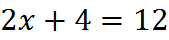We subtract 4 from both sides: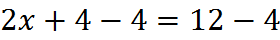Then we combine terms to get: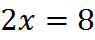Finally, we divide by the coefficient of x: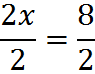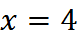When you’re given more difficult equations, the sure way to isolate a variable is to perform the following steps:

1. Eliminate any fractions present by multiplying both sides by any denominators present.

2. Get all terms with the variable on the left side of the equation, and all terms without the variable on the other side.

3. Combine the terms on the left side, if possible.

4. Factor out the variable on the left side, if necessary.

5. Divide both sides by the coefficient of the variable, leaving the variable isolated.

At five steps, this process might seem laborious. If you remember to eliminate fractions first and divide last, that’s a good start. For this reason and since isolating is a common procedure on the GMAT, a good way to practice is to dive right into some realistic questions.

## Practice Questions

Variable in a Denominator:
http://www.gmatfree.com/variable-in-a-denominator

Needless Variable:
http://www.gmatfree.com/needless-variable

Linear Equations with Fractions:
http://www.gmatfree.com/linear-equations-with-fractions

Welcome! You are encouraged to register with the site and login (for free). When you register, you support the site and your question history is saved.